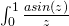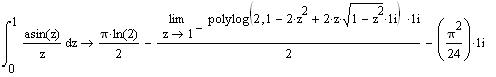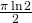## A trickly little integral.

December 10th, 2007 | Categories: general math, math software | Tags:

I was recently playing around with version 14 of Mathcad and discovered that it had a problem with calculating the following indefinite integral symbolically.Which looks pretty scary. If I bypass the symbolic engine and calculate it numerically then I get a result of about 1.089 which is equal to the first term of Mathcad’s symbolic solution:So if MathCAD has got the symbolic solution correct then the scary looking term containing the limit should cancel out the imaginary term. I have never come across the polylog function before so I tried to look it up in Mathcad’s help system but there was no reference to it there – a bit of an oversight in my opinion considering it seems to be a built in function. (A google search later and polylog turns out to be short for polylogarithm – the entry on Wolfram’s mathworld looks like a good place to start learning about it – yet another thing on my list of things to learn.)

Clearly Mathcad’s symbolic engine wasn’t capable of evaluating this limit so I had to try something else. The thought of trying to evaluate it by hand briefly crossed my mind but I didn’t fancy my chances much since I didn’t have a clue about the polylog function. My next thought was to fire up Matlab (2007a) and try its symbolic toolbox. First of all – Would it succeed to evaluate the integral where Mathcad had failed?

>> syms x
>> int(asin(x)/x,x,0,1)
ans =1/8*i*pi^2+1/4*pi*log(2)+1/2*pi*log(1-i)
>> simplify(ans)
ans =1/2*pi*log(2)

No problems there then but could it help with the polylog limit that would determine if I had found a bug in Mathcad? At first, the answer seemed to be ‘no’ since I couldn’t find a reference to polylog in Matlab either but, after a bit more googling, I discovered that polylog(2,x) is sometimes called the dilogarithm function and this is implemented in matlab as dilog(x) according to the help browser. Unfortunately it is not implemented for symbolic variables so I was stumped there as well.

Since I am a fortunate soul and have access to pretty much every computer algebra system that is worth having I then turned to Mathematica. Just like Matlab, Mathematica had no problem with solving the integral that stumped Mathcad:

Integrate[ArcSin[x].{x,0,1}]

gave

1/2*Pi*Log

It also fully implemented the polylog function so I could try the limit:

-I/2*Limit[PolyLog[2, 1 – 2 x^2 + 2 x Sqrt[1 – x^2]], x -> 1]

gave

-I Pi^2/24

Which confirmed that Mathcad had got the correct result but just couldn’t simplify it fully like its two rivals could.

Finally, I would like to set a challenge to anyone who stumbles across this little part of the web – could you evaluate this integral by hand? I can’t but would love to know how it might be done. If anyone finds a solution please let me know.

1. When you use Mathcad to analytically evaluate that integral, you need to use limit before the integral (the integrand approaches 1 as z goes to 0). For this particular case, two-sided or right-hand limit will do. You will get exactly what you want.